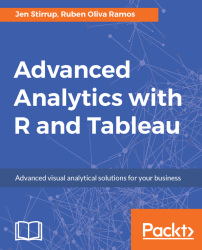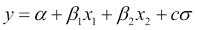•#### Advanced Analytics with R and Tableau#### Overview of this book

Tableau and R offer accessible analytics by allowing a combination of easy-to-use data visualization along with industry-standard, robust statistical computation. Moving from data visualization into deeper, more advanced analytics? This book will intensify data skills for data viz-savvy users who want to move into analytics and data science in order to enhance their businesses by harnessing the analytical power of R and the stunning visualization capabilities of Tableau. Readers will come across a wide range of machine learning algorithms and learn how descriptive, prescriptive, predictive, and visually appealing analytical solutions can be designed with R and Tableau. In order to maximize learning, hands-on examples will ease the transition from being a data-savvy user to a data analyst using sound statistical tools to perform advanced analytics. By the end of this book, you will get to grips with advanced calculations in R and Tableau for analytics and prediction with the help of use cases and hands-on examples.
Advanced Analytics with R and TableauCreditswww.PacktPub.comCustomer FeedbackPrefaceFree Chapter
Advanced Analytics with R and TableauThe Power of RA Methodology for Advanced Analytics Using Tableau and RPrediction with R and Tableau Using RegressionClassifying Data with TableauIndex## Bayesian Theory

We can implement Bayesian probability using Python. For our demo, we generate output values from two independent variables, x1 and x2. The output model is defined as follows:c is a random value. We define α, β1, β2, and σ as 0.5, 1, 2.5, and 0.5.

These independent variables are generated using a random object from the `NumPy` library. After that, we compute the model with these variables.

We can implement this case with the following scripts:

```import matplotlib
matplotlib.use('Agg')
import numpy as np
import matplotlib.pyplot as plt
# initialization
np.random.seed(100)
alpha, sigma = 0.5, 0.5
beta = [1, 2.5]
size = 100

# Predictor variable
X1 = np.random.randn(size)
X2 = np.random.randn(size) * 0.37
# Simulate outcome variable
Y = alpha + beta*X1 + beta*X2 + np.random.randn(size)*sigma
fig, ax = plt.subplots(1, 2, sharex=True, figsize=(10, 4))